CATEGORIES:

# Trigonometric form of a complex number, de Moivre's formula

Use the above figure to express the real and imaginary parts a,b

of the complex number in terms of the magnitude and argument ρ, j.

a=r.cosj, b=r.sinj.

Hence

a=r(cosj+isinj)

This is the trigonometric form of a complex number.

Each complex number has a unique trigonometric form because it has a unique magnitude and argument.

Example. α=.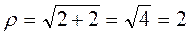;.

Therefore,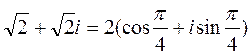.

1. Multiplication. Consider complex numbers

a1 =r1 (cosj1 +isinj1) and a2 =r2 (cosj2 +isinj2).

The product equals

a1·a2 = r1 (cosj1 +isinj1). r2 (cosj2 +isinj2)=

=r1·r2 [(cosj1cosj2 -sinj1sinj2)+i(sinj1cosj2 + cosj1sinj2);

a1·a2 = r1·r2 [cos(j1+j2 )+isin(j1+j2 )].

Therefore, in order to multiply two complex numbers, it is necessary to multiply their magnitudes and add the arguments.

2.Division. Multiply both the numerator and denominator by the conjugate of the denominatorTherefore, in order to divide two complex numbers, it is necessary to divide their magnitudes and subtract the arguments.

A power of a complex number. If a1=a2=a3=…=an=a, then

an = r ·r ·r·…·r[cos(j+j +j+…+j)+i(sin(j+j +j+…+j)]= =rn[cosnj+isinnj]

or

ak =rk[coskj+isinkj] - de Moivre's formula.

Roots of a complex number. Write down de Moivre's formula for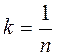using the fact the sine and cosine are periodic functions with the period T=2p: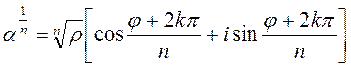,

where k may be any of n integers 0,1,2,…,n-1.

Example. Solve the equation x3+1=0.

1-st method: (x+1)(x2-x+1)=0 , x1=-1,;

2-nd method: x3=-1,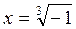, -1=cosp+isinp, which is a trigonometric form of a number.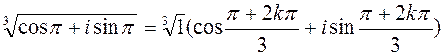. Using the formula, k=0,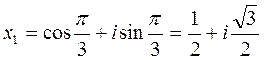,

k=1,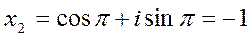,

k=2,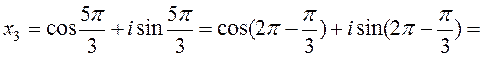=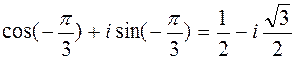.

ELABORATIONS OF PRACTICAL CLASSES

Date: 2015-01-02; view: 1487

 <== previous page | next page ==> COMPLEX NUMBER. COMPLEX NUMBERS IN TRIGONOMETRIC AND EXPONENTIAL FORM | Matrix. Operations with matrices. The inverse matrix. Determinants and their properties. Systems of linear equations
doclecture.net - lectures - 2014-2023 year. Copyright infringement or personal data (0.006 sec.)